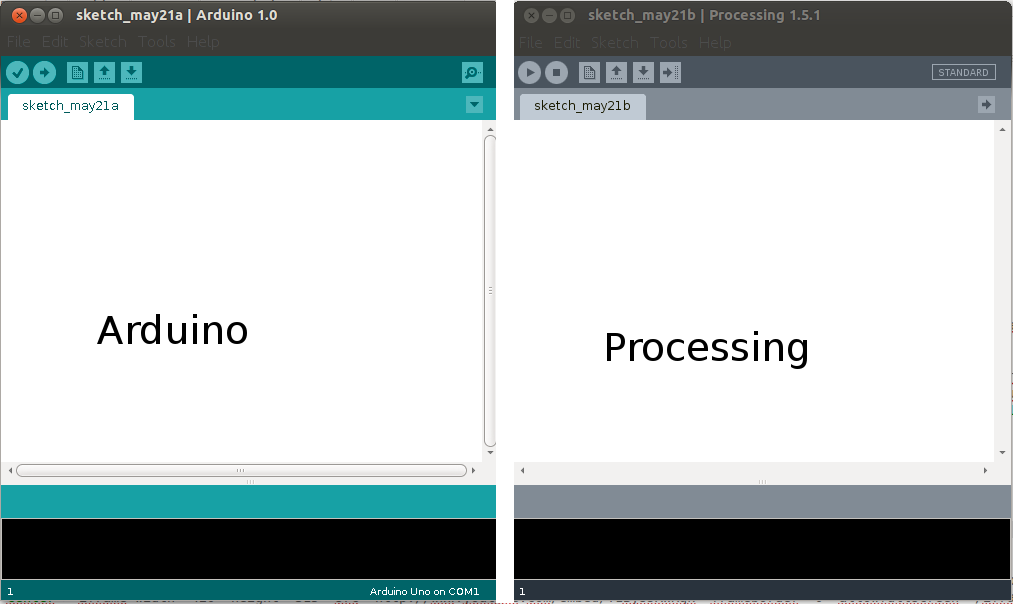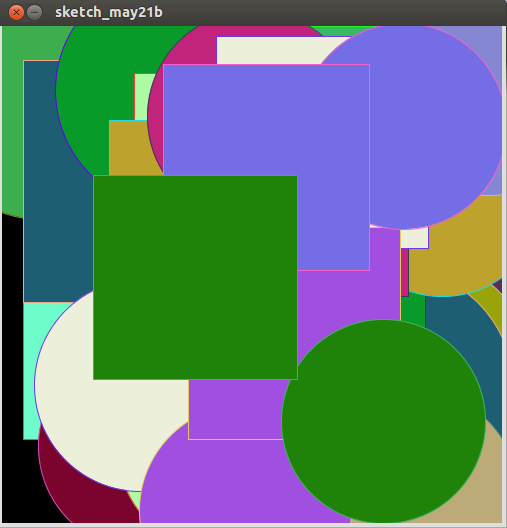# Author's Note: I'm back!!

This assignment is of development in Processing,so that this assignment consists of:

--Why use processing?
--Programming in Processing

Why use Processing?

In the previous assignment "inputs", was done in python, but in my opinion,the team did not understand the lines of code. Investigated with respecto to means each line of code, but without sucess. subsequently observed Providence tutotials that make use of Processing, investigated again and got four conclusions:

--It is of the same family as Arduino
--It is quite intuitive and specific for visual development
--There is documentation related to my final project
--Does not have installation problems on Ubuntu 4.12

Here I show interfaces Arduino and Processing.This will reinforce my learning Arduino
If you want to install Processing click here
Aquí You can find manuals and applications.

Programming in Processing

After reading some documentation and sample applications, I took the code provided in the tutorial chapter.
I use the sketch 2, I made ​​two changes:

Modify the starting point in the coordinate (250,250) making a random start, modify the background, But I was not so sure:
The background code is: Add in void setup() = int b= 10; y en void draw() b=b+1; background(b)
but the result is not optimal, finally only modify the ellipse: ellipse(random(500),random(500), diameter, diameter); also add a rectangle: rect(random(500),random(500), diameter, diameter)

/**
* Phototransistor Input to Circle
*
* Read data from the serial port and changes the fill and stroke color of a circle
* relative to the values recieved from the sensor.
* Circle fill and stroke are random, unless a sensor value below 200 is recived.
*/

//import serial library
import processing.serial.*;

Serial serialPort; // Create object from Serial class
int diameter= 500; // initial diameter of the circle

void setup() {
size(500, 500);

stroke(255);
smooth();
// I know that the first port in the serial list on my mac
// is always my FTDI adaptor, so I open Serial.list().
// On Windows machines, this generally opens COM1.
// Open whatever port is the one you're using.
println(Serial.list());
serialPort = new Serial(this, Serial.list(), 9600);
}

void draw() {
//filter out the 1,2,3,4 framing code numbers
while (serialPort.available () > 4) {
int lightIn = serialPort.read();
//if the sensor value is greater than 200
//(you may need to tweak this number to get the code to respond to your board)

if (lightIn > 200) {
//print in the sensor values to the console
println(lightIn);
//set the variable diameter to the sensor value
diameter = lightIn;
//use the sensor value to draw an ellipse
ellipse(random(500), random(500), diameter, diameter);
rect(random(500), random(500), diameter, diameter);

//redraw the screen
redraw();
}

}
//EXPERIMENT WITH THE VISUALIZATION BELOW
//if the sensor values are less that 200, the elipse with random stroke and fill colors
stroke((int)random(255), (int)random(255), (int)random(255));
fill((int)random(255), (int)random(255), (int)random(255));
}

The final result is the following image an video: